Case Based Questions (MCQ)

Chapter 1 Class 10 Real Numbers
Serial order wise

## A Mathematics Exhibition is being conducted in your School and one of your friends is making a model of a factor tree. He has some difficulty and asks for your help in completing a quiz for the audience. Observe the following factor tree and answer the following: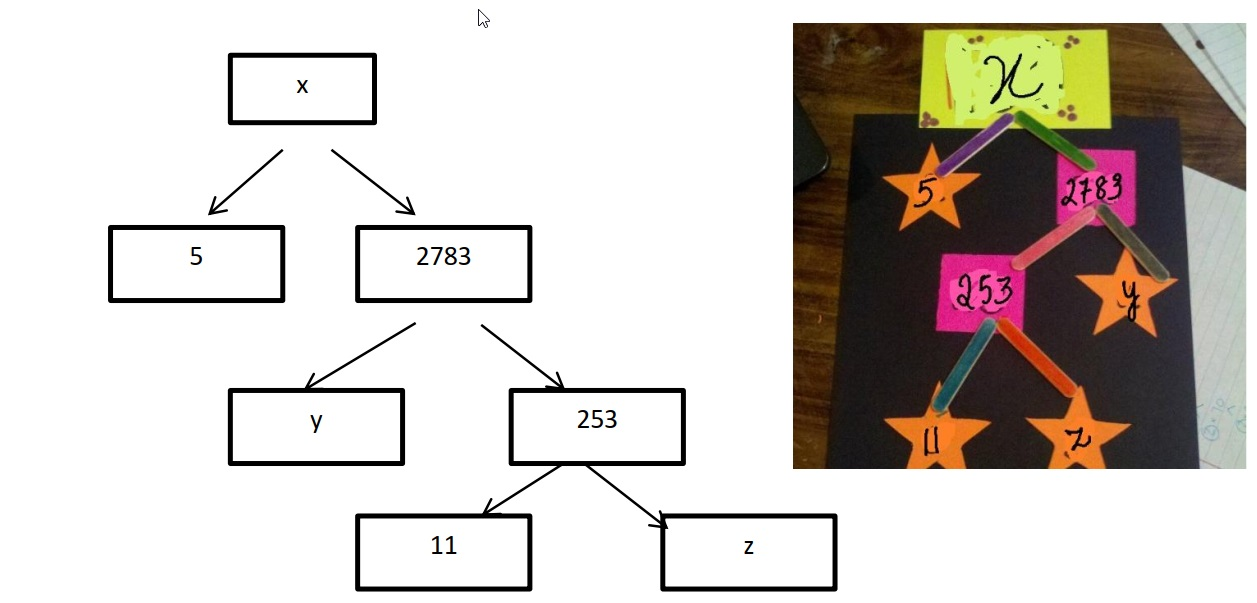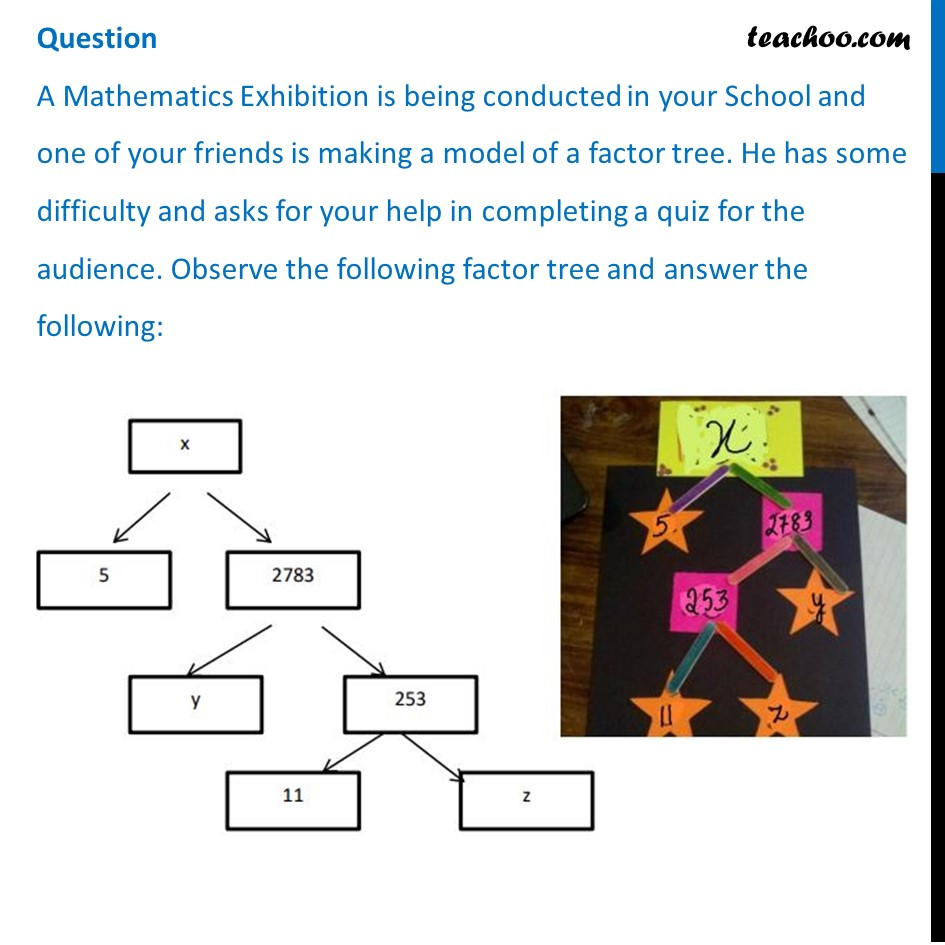## What will be the value of x? (a) 15005   (b) 13915  (c) 56920  (d) 17429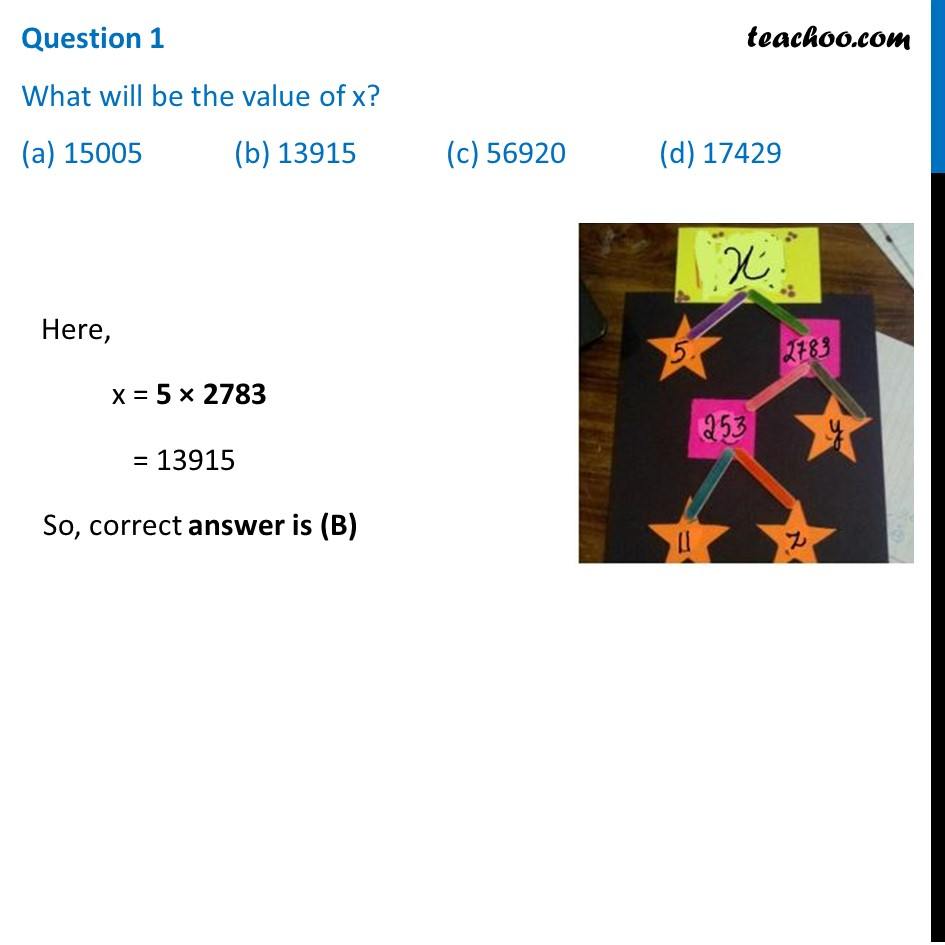## What will be the value of y? (a) 23  (b) 22  (c) 11  (d) 19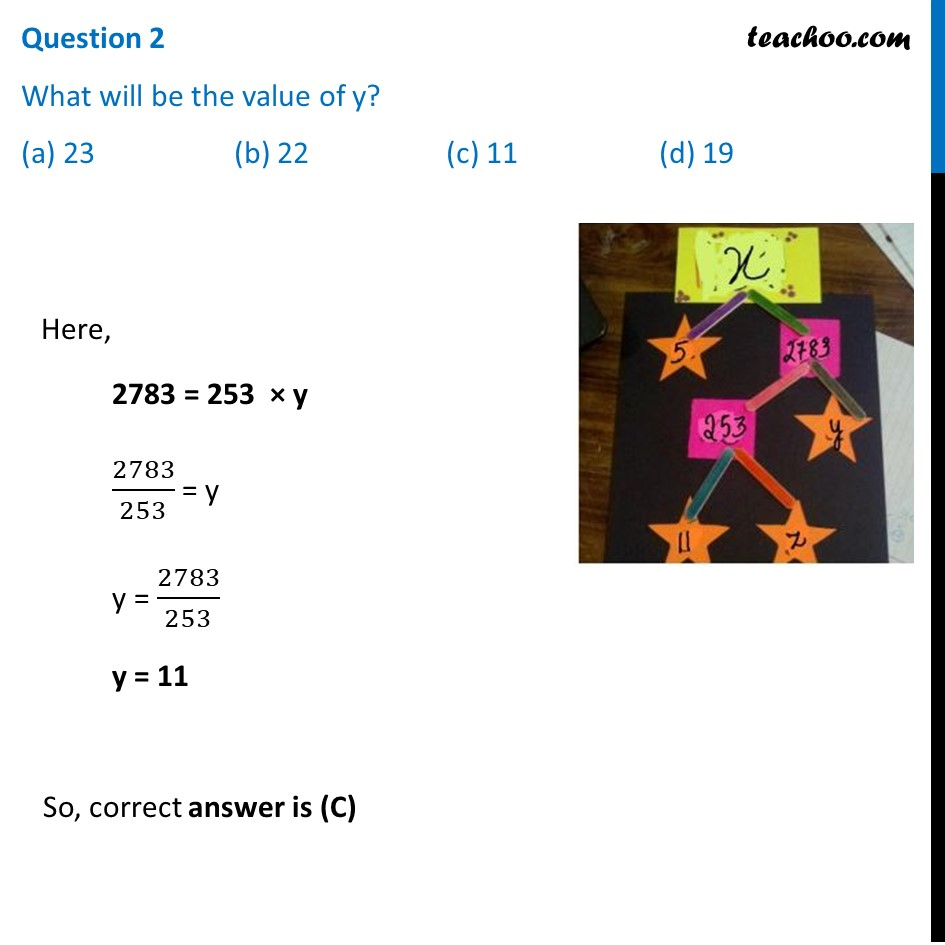## What will be the value of z? (a) 22  (b) 23  (c) 17  (d) 19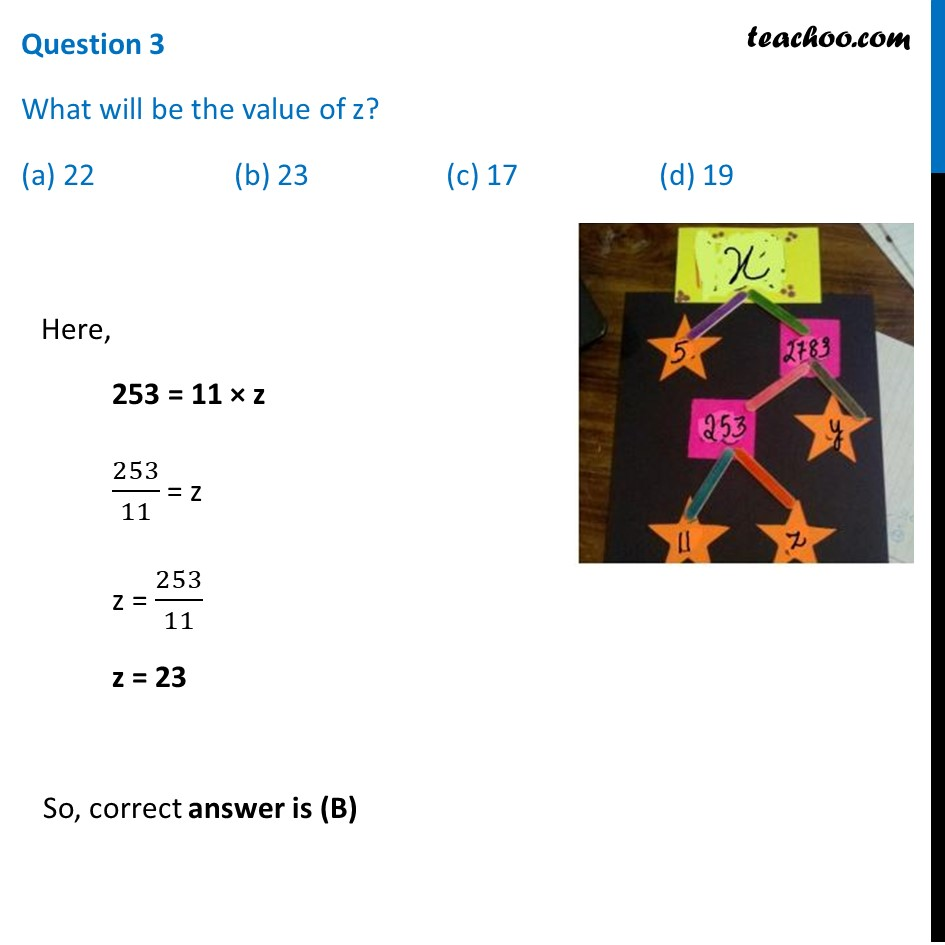## According to Fundamental Theorem of Arithmetic 13915 is a (a) Composite number   (b) Prime number   (c) Neither prime nor composite   (d) Even number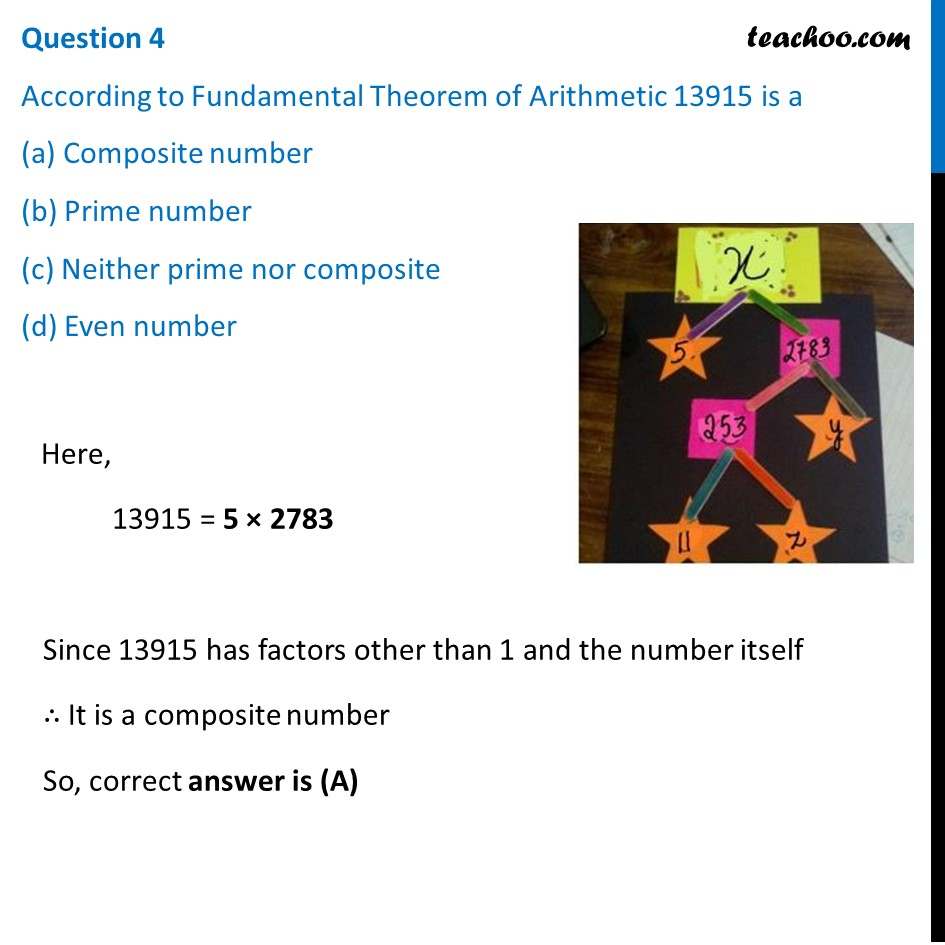## The prime factorisation of 13915 is (a) 5 × 11 3   × 13 2 (b) 5 × 11 3 × 23 2 (c) 5 × 11 2 × 23  (d) 5 × 11 2 × 13 2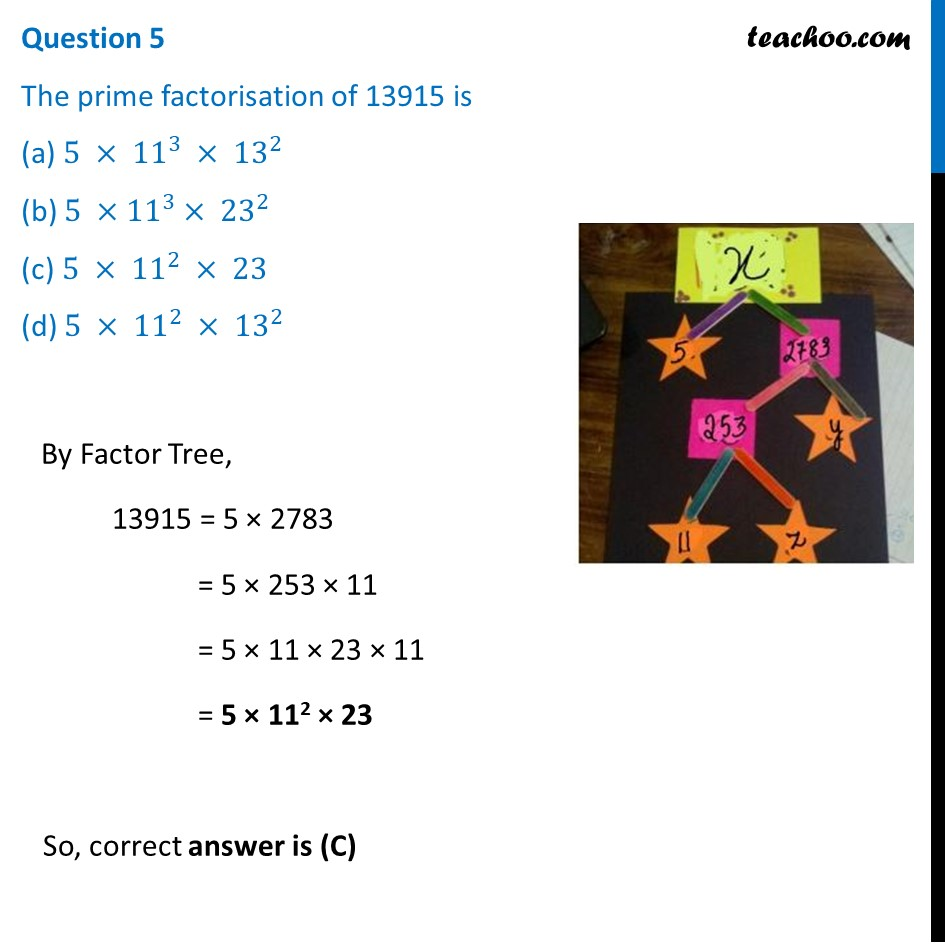Learn in your speed, with individual attention - Teachoo Maths 1-on-1 Class

### Transcript

Question A Mathematics Exhibition is being conducted in your School and one of your friends is making a model of a factor tree. He has some difficulty and asks for your help in completing a quiz for the audience. Observe the following factor tree and answer the following:Question 1 What will be the value of x? (a) 15005 (b) 13915 (c) 56920 (d) 17429Here, x = 5 × 2783 = 13915 So, correct answer is (B) Question 2 What will be the value of y? (a) 23 (b) 22 (c) 11 (d) 19 Here, 2783 = 253 × y 2783/253 = y y = 2783/253 y = 11 So, correct answer is (C) Question 3 What will be the value of z? (a) 22 (b) 23 (c) 17 (d) 19 Here, 253 = 11 × z 253/11 = z z = 253/11 z = 23 So, correct answer is (B) Question 4 According to Fundamental Theorem of Arithmetic 13915 is a (a) Composite number (b) Prime number (c) Neither prime nor composite (d) Even number Here, 13915 = 5 × 2783 Since 13915 has factors other than 1 and the number itself ∴ It is a composite number So, correct answer is (A) Question 5 The prime factorisation of 13915 is (a) 5 × 11^3 × 13^2 (b) 5 ×〖 11〗^3 × 23^2 (c) 5 × 11^2 × 23 (d) 5 × 11^2 × 13^2 By Factor Tree, 13915 = 5 × 2783 = 5 × 253 × 11 = 5 × 11 × 23 × 11 = 5 × 112 × 23 So, correct answer is (C)